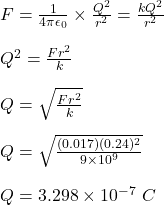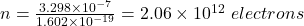## A charge Q is transferred from an initially uncharged plastic ball to an identical ball 24 cm away.The force of attraction is then 17 mN. Ho

Question

A charge Q is transferred from an initially uncharged plastic ball to an identical ball 24 cm away.The force of attraction is then 17 mN. How many electrons were transferred from one ball to the other?

in progress 0
3 months 2021-07-25T17:35:05+00:00 1 Answers 16 views 0

The number of electrons transferred from one ball to the other is 2.06 x 10¹² electrons

Explanation:

Given;

magnitude of the attractive force, F = 17 mN = 0.017 N

distance between the two objects, r = 24 cm = 0.24 m

The attractive force is given by Coulomb’s law;The charge of 1 electron = 1.602 x 10⁻¹⁹ C

n(1.602 x 10⁻¹⁹ C) = 3.298 x 10⁻⁷Therefore, the number of electrons transferred from one ball to the other is 2.06 x 10¹² electrons